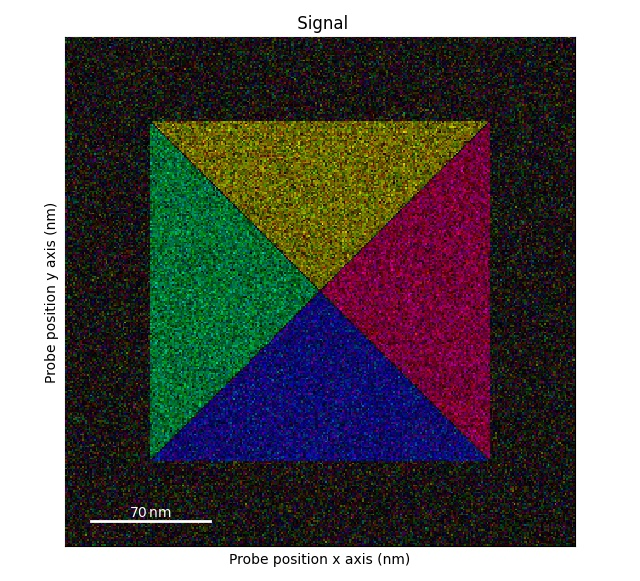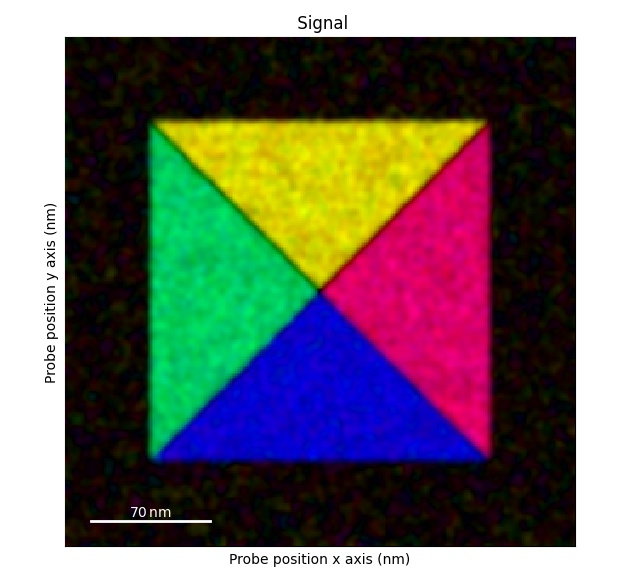# Analysing differential phase contrast data¶

Example of a paper doing this type of data processing: Strain Anisotropy and Magnetic Domains in Embedded Nanomagnets. The data and processing scripts used in this paper is available at Zenodo with the DOI 10.5281/zenodo.3466591.

Differential phase contrast analysis (DPC) is done using using the DPCSignal classes: `DPCSignal2D`, `DPCSignal1D` and `DPCBaseSignal`.

Here, a test dataset is used to show the methods used to process this type of data. For information on how to load your own data, see :ref:load_dpc_data.

```>>> import pixstem.api as ps
>>> s.plot()
```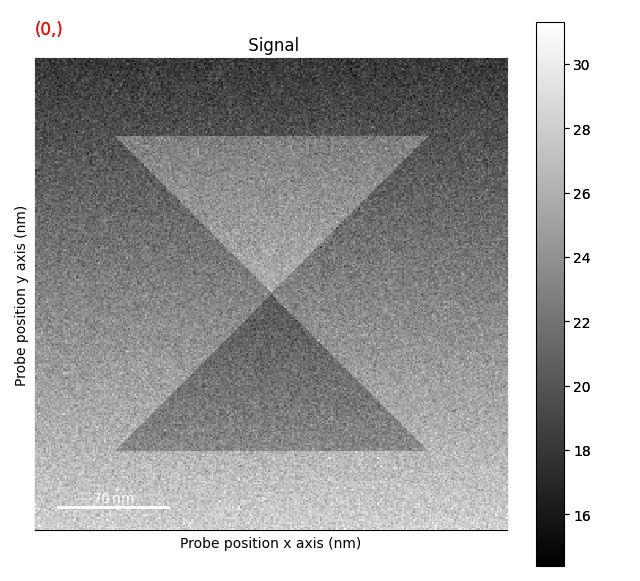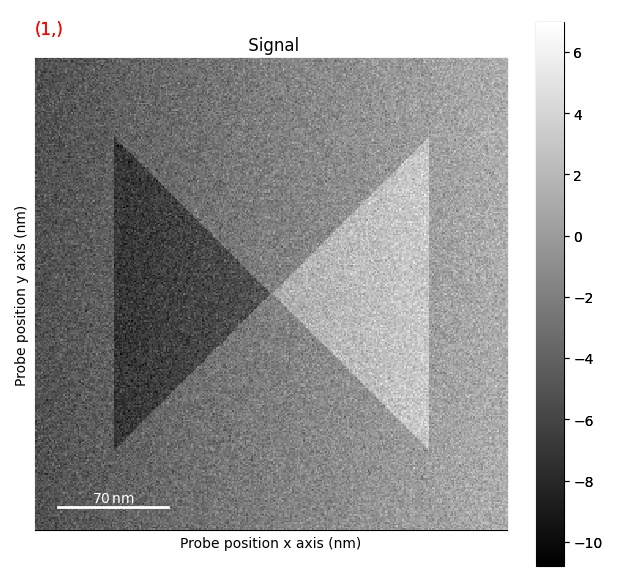## Correcting d-scan¶

`correct_ramp()`

The first step is the remove the effects of the d-scan:

```>>> s = s.correct_ramp()
>>> s.plot()
```

Note that this method only does a plane correction, using the corners of the signal. For many datasets, this will not work very well. Possibly tweaks is to change the corner size.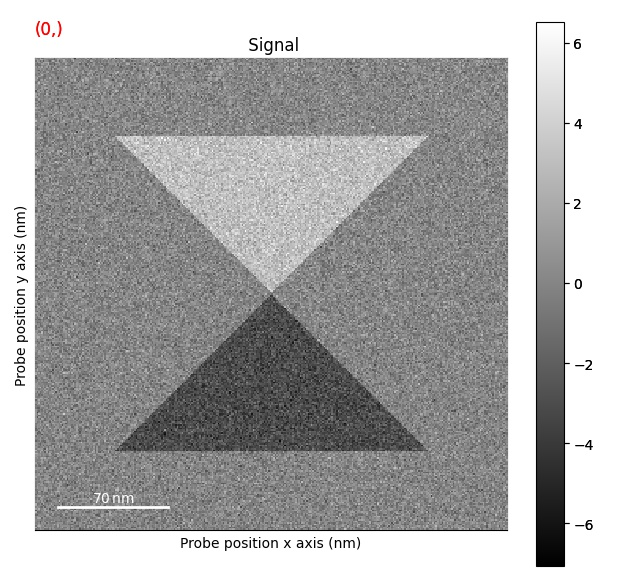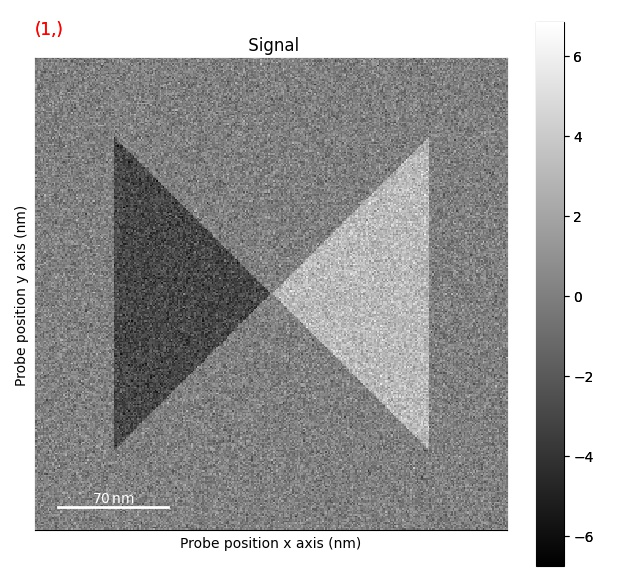## Plotting methods¶

Plotting DPC color image using: `get_color_signal()`

```>>> s_color = s.get_color_signal()
>>> s_color.plot()
```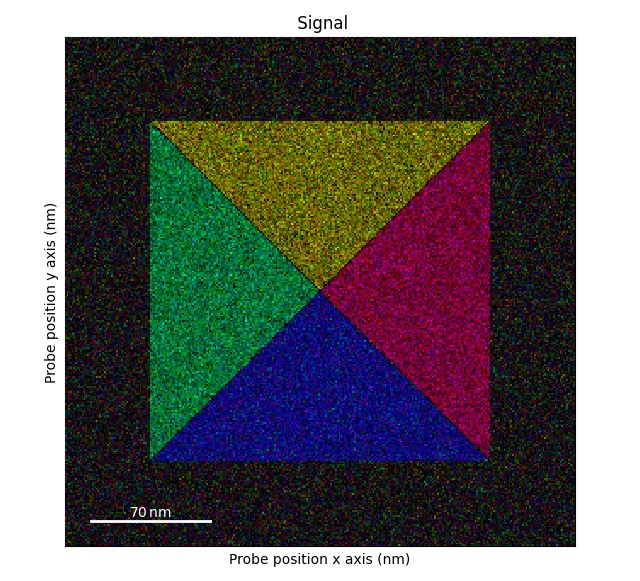Plotting DPC phase image: `get_phase_signal()`

```>>> s_phase = s.get_phase_signal()
>>> s_phase.plot()
```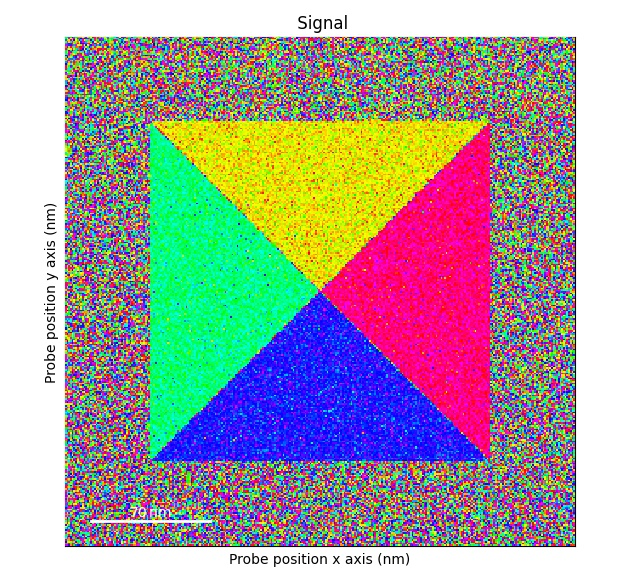Plotting DPC magnitude image: `get_magnitude_signal()`

```>>> s_magnitude = s.get_magnitude_signal()
>>> s_magnitude.plot()
```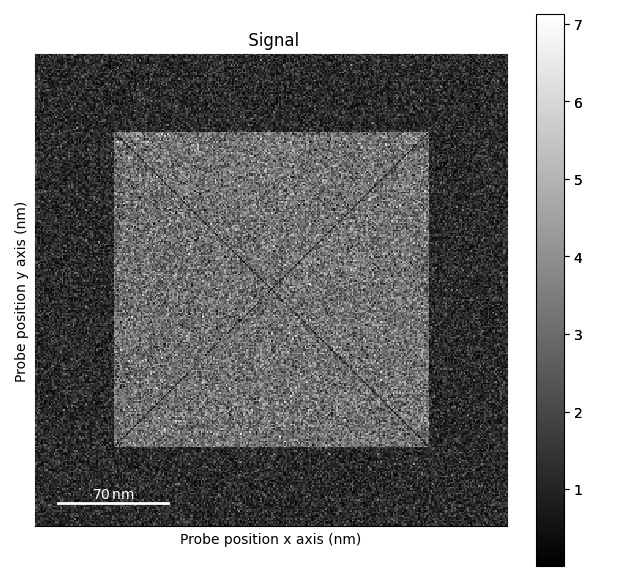Plotting bivariate histogram: `get_bivariate_histogram()`

```>>> s_hist = s.get_bivariate_histogram()
>>> s_hist.plot(cmap='viridis')
```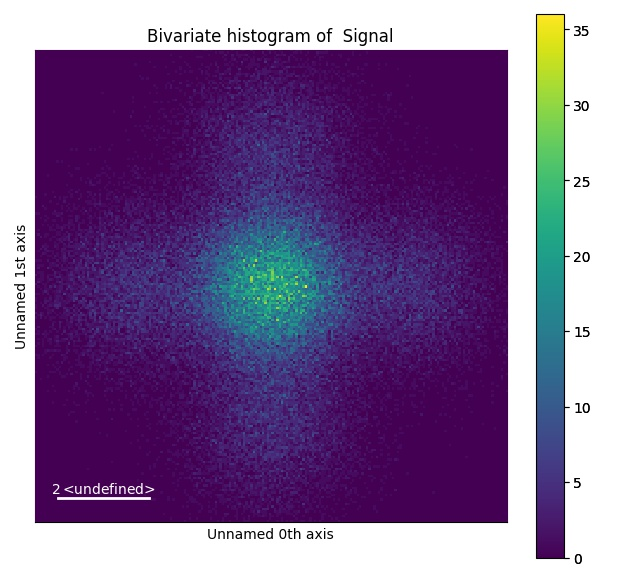Plotting color image with more customizability: `get_color_image_with_indicator()`

```>>> fig = s.get_color_image_with_indicator()
>>> fig.show()
```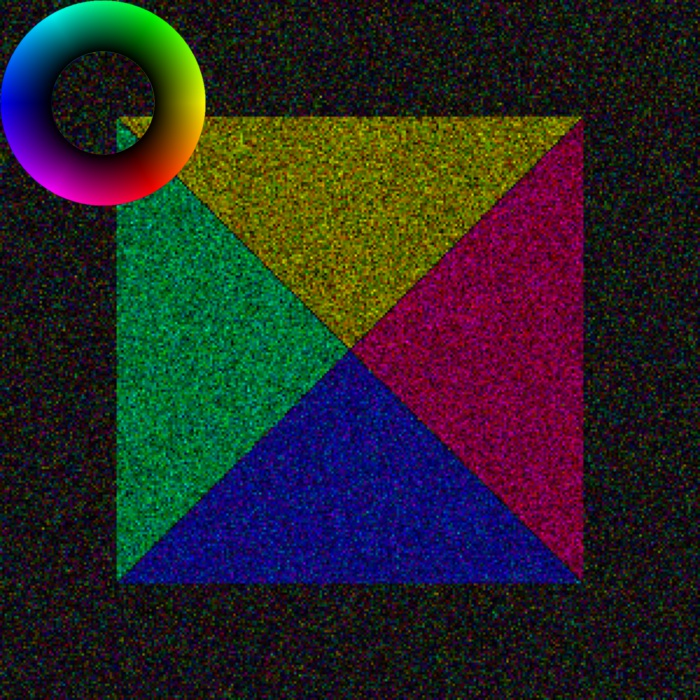## Rotating the data¶

Rotating the probe axes: `rotate_data()`. Note, this will not rotate the beam shifts.

```>>> s_rot_probe = s.rotate_data(10)
>>> s_rot_probe.get_color_signal().plot()
```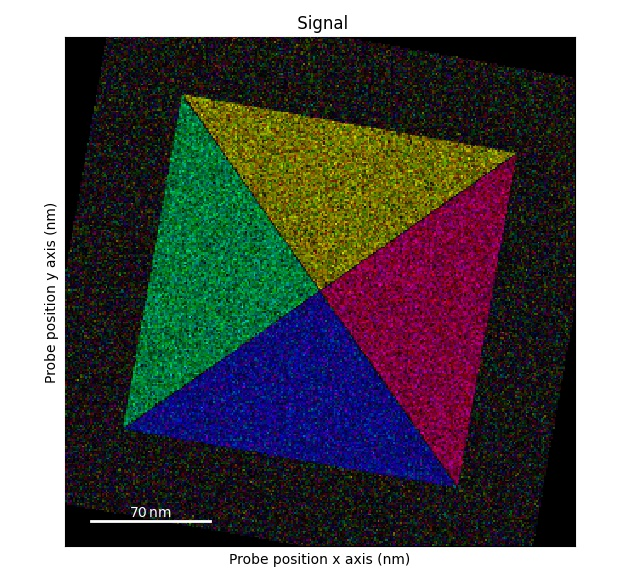Rotating the beam shifts: `rotate_beam_shifts()`.

```>>> s_rot_shifts = s.rotate_beam_shifts(45)
>>> s_rot_shifts.get_color_signal().plot()
```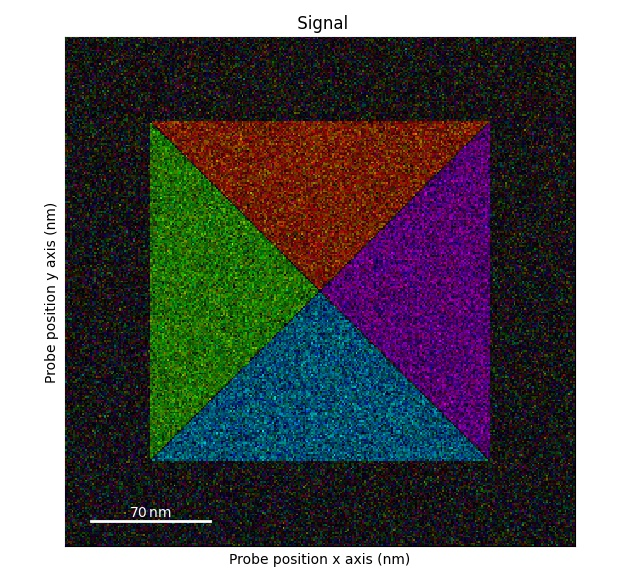Rotating both the probe dimensions and beam shifts by 90 degrees: `flip_axis_90_degrees()`. Note: in this dataset there will not be any difference compared to the original dataset. So we slightly alter the dataset.

```>>> s1 = s.deepcopy()
>>> s1.data[0, 50:250, 145:155] += 5
>>> s1.get_color_signal().plot()
>>> s_flip_rot = s1.flip_axis_90_degrees()
>>> s_flip_rot.get_color_signal().plot()
```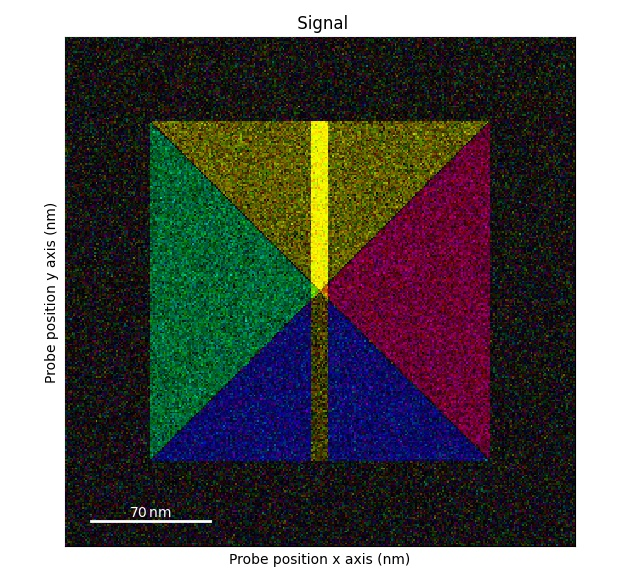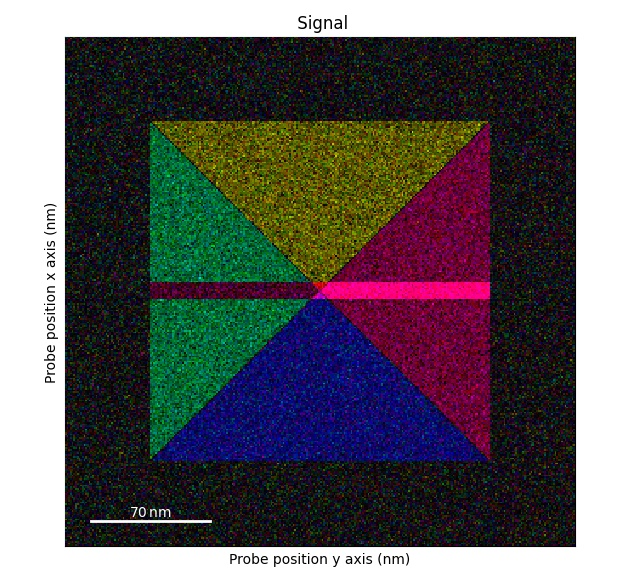## Blurring the data¶

The beam shifts can be blurred using `gaussian_blur()`.

This is useful for suppressing the effects of variations in the crystal structure.

```>>> s = ps.dummy_data.get_square_dpc_signal()
>>> s_blur = s.gaussian_blur()
>>> s.get_color_signal().plot()
>>> s_blur.get_color_signal().plot()
```